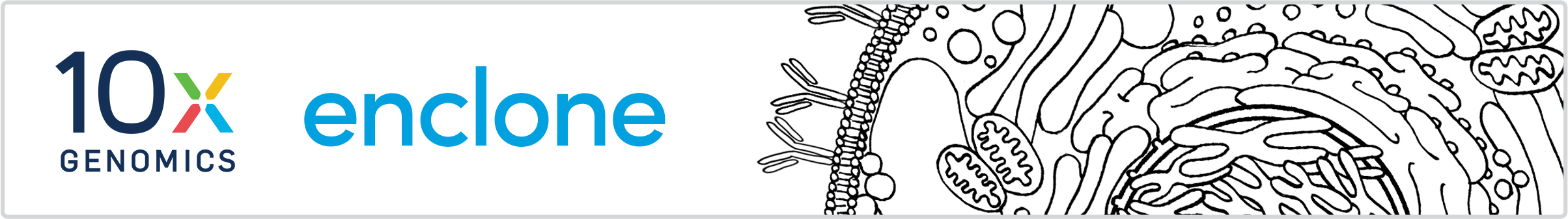# enclone heuristics

Clonotype chain grouping. After exact subclonotypes have been grouped into clonotypes, we decide which chains from which exact subclonotypes are placed in the same column of the table for the clonotype. While in most particular instances the answer is "obvious", the general problem is complicated. We proceed by "joining" chains, i.e. deciding that they will go in the same column. There are several steps:

1. At the earlier point in the algorithm where we decide that two exact subclonotypes go in the same clonotype, we align a heavy or TRB chain from each (one from each exact subclonotype) to the other, and likewise for the light or TRA chains. This defines a correspondence between chains, and at the subsequent point when we generate clonotype tables, this information is carried forward to join chains.
2. The initial process misses some joins for two reasons: (1) because in the initial join step, for computational performance reasons, we only test as many joins as are needed to form the clonotypes, so some joins are not seen, and this is compounded by filtering steps that delete putatively artifactual exact subclonotypes; (2) when we join two exact subclonotypes, and one or both have three chains, we stop looking once we've joined them and thus do not look at all the chains. To mitigate these two problems, at the time of forming clonotype tables, we recover some of the "lost" joins.
3. In the special case where two exact subclonotypes are joined, and both have three chains, we apply a lower threshold for merging the "third" chain. This threshold is that the V..J sequences have the same length and differ at at most 10 bases.
4. We also connect onesie exact subclonotypes to other chains by matching based on exact identity of V..J.
This description is accurate for the current enclone, and corresponds to changes that will appear in Cell Ranger in a version after 6.0.

Exact subclonotype ordering within a clonotype. Originally we tried ordering the exact clonotypes in reverse order by the number of cells in a given exact subclonotype. This had the perverse effect of sometimes placing onesie (single chain) clonotypes at the top, which we thought not helpful. After some experimentation we arrived at the following ordering, which although arbitrary, tends to place "more interesting" exact subclonotypes near the top:

1. For each exact subclonotype, we form a vector of boolean values reflecting the presence of a given chain in an exact subclonotype. For example, in a two-chain clonotype, if both chains are present, the vector is `(true, true)`. The ordering is reverse lexicographical, so that in the two chain case, the ordering is:
````(true, true)`
`(true, false)`
`(false, true)`.```
2. Subject to this, exact subclonotypes are then reverse ordered by number of cells.
3. Subject to the first two orderings, exact subclonotypes are then reverse ordered by the total number of UMIs, summed across chains.